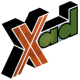-=+=- -=+=- -=+=- -=+=- -=+=- -=+=- -=+=- -=+=- -=+=- -=+=- -=+=- -=+=- -=+=- -=+=- -=+=- -=+=- -=+=- -=+=- -=+=- -=+=- -=+=- -=+=- -=+=- -=+=- -=+=- -=+=- -=+=- -=+=- -=+=- -=+=- (c) WidthPadding Industries 1987 0|544|0 -=+=- -=+=- -=+=- -=+=- -=+=- -=+=- -=+=- -=+=- -=+=- -=+=- -=+=- -=+=- -=+=- -=+=- -=+=- -=+=- -=+=- -=+=- -=+=- -=+=- -=+=- -=+=- -=+=- -=+=- -=+=- -=+=- -=+=- -=+=- -=+=- -=+=- Socoder -> Off Topic -> The Extreme Topic of Indefinability Posted : Sunday, 18 August 2013, 18:00 JayenkaiSocoder is a programming forum for people who create things. Socoder is not a friend-code whorehouse. -=-=- ''Load, Next List!''Posted : Sunday, 18 August 2013, 18:00 dnaWhat is a Friend Code Whorehouse? Posted : Sunday, 18 August 2013, 19:21 CodersRule*poke*Posted : Sunday, 18 August 2013, 19:59 steve_ancellen.wikipedia.org/wiki/Nintendo_Wi-Fi_Connection#Friend_Codes In Laymen's terms, a Friend Code Whorehouse is a place for exchanging Nintendo IDs, AKA finding other Nintendo network users. Posted : Sunday, 18 August 2013, 20:28 9572AD...and here I was hoping it was a place where you could pay in Friend Codes. -=-=- All the raw, animal magnetism of a rutabaga.